# Spatial Data Retrieval API¶

Spatial values retrieved from the database are represented by special classes included into the SDK. Consider the following example - the `Person` table contains the `location` column of type `POINT:`The code sample below retrieves the first object from the table and accesses the value from the column. Notice the value is represented by an object of class `Point`:

Person class

``````@interface Person : NSObject

@property (strong, nonatomic) BLPoint *location;

@end
``````
Code to fetch a person object with spatial data
``````[[Backendless.shared.data of:[Person class]] findFirstWithResponseHandler:^(Person *person) {
BLPoint *location = person.location;
double locationLatitude = location.latitude;
double locationLongitude = location.longitude;
} errorHandler:^(Fault *fault) {
NSLog(@"Error: %@", fault.message);
}];
``````

Person class

``````@objcMembers class Person: NSObject {
var location: BLPoint?
}
``````
Code to fetch a person object with spatial data
``````Backendless.shared.data.of(Person.self).findFirst(responseHandler: { person in
guard let person = person as? Person else { return }
let location = person.location
let locationLatitude = location?.latitude
let locationLongitude = location?.longitude
}, errorHandler: { fault in
print("Error: \(fault.message ?? "")")
})
``````

``````[[Backendless.shared.data ofTable:@"Person"] findFirstWithResponseHandler:^(NSDictionary *person) {
BLPoint *location = person[@"location"];
double locationLatitude = location.latitude;
double locationLongitude = location.longitude;
} errorHandler:^(Fault *fault) {
NSLog(@"Error: %@", fault.message);
}];
``````
``````Backendless.shared.data.ofTable("Person").findFirst(responseHandler: { person in
let location = person["location"] as? BLPoint
let locationLatitude = location?.latitude
let locationLongitude = location?.longitude
}, errorHandler: { fault in
print("Error: \(fault.message ?? "")")
})
``````

As a general rule, when retrieving data from the Backendless database, client SDK converts values as shown below:

column type
value is returned as an instance of
`POINT`
`BLPoint`
`LINESTRING`
`BLLineString`
`POLYGON`
`BLPolygon`

## Where Clause Operators¶

A where clause is a condition used in the data retrieval API. The condition identifies the objects which should be retrieved from the database. There are several specialized where clause operators which work specifically with the columns of the spatial data types.

### Within Operator¶

This operator retrieves all data objects whose values for the specified spatial type column are within the boundary of the specified geometry.

Syntax:

``````Within( spatialColumnName, 'Geometry as WKT' ) = Condition
``````

where:

Argument                Description
`spatialColumnName` Name of a spatial type column in the data table where the data objects are retrieved from; Returned objects have values in the `spatialColumnName` column which are within the geometry identified by `'Geometry as WKT'`.
`'Geometry as WKT'` A WKT string (must be enclosed into single quotes) which designates the boundary of a shape where Backendless will search for values from the `spatialColumnName` column;
`Condition` `True` or `False`

Examples:

Retrieve all data objects where the geometries in the `location` column are within the specified polygon:

``````Within(location, 'POLYGON((-154.53617745476788 7.820063820687473, -154.53617745476788 51.13813853838118, -14.87797432976788 51.13813853838118, -14.87797432976788 7.820063820687473, -154.53617745476788 7.820063820687473))') = True
``````

Assuming the `location` column contains `POINT` values, retrieve all data objects where the points in location are the same as the specified WKT point:

``````Within(location, 'POINT (-96.77016753 32.82433675)') = True
``````

Codeless Reference

Consider the following records and locations in the `Towns` data table: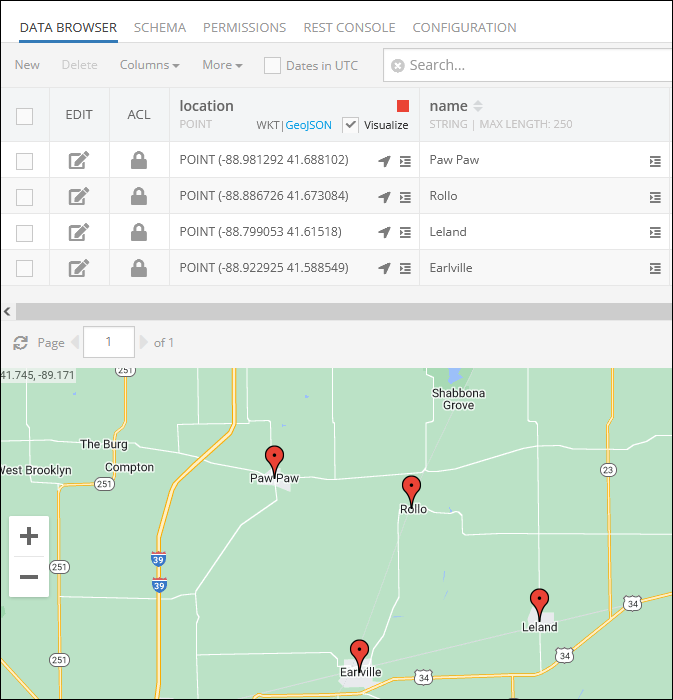The example below retrieves all data objects where the geometries in the `location` column are within the specified polygon:

``````Within(location, 'POLYGON((-88.981058 41.713514, -88.850866 41.679702, -88.873037 41.647986, -89.015021 41.686395, -88.981058 41.713514))') = True
``````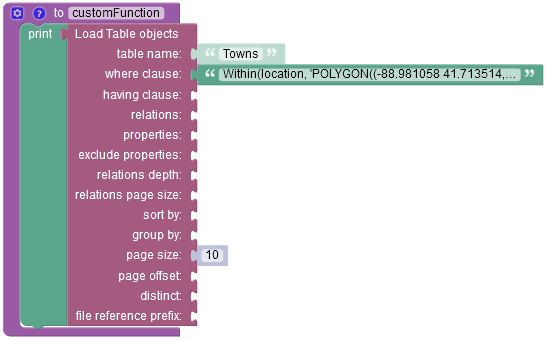The result of this operation will look as shown below after the Codeless logic runs. As you can see, the operation has returned two objects whose Points(coordinates) are within the geometry of the specified polygon.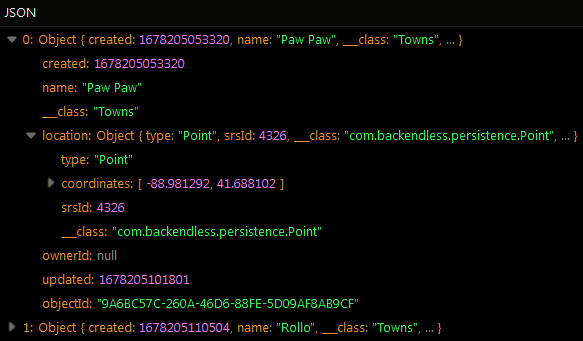Suppose you need to retrieve all data objects where the points in location are the same as the specified WKT point:

``````Within(location, 'POINT (-88.886726 41.673084)') = True
``````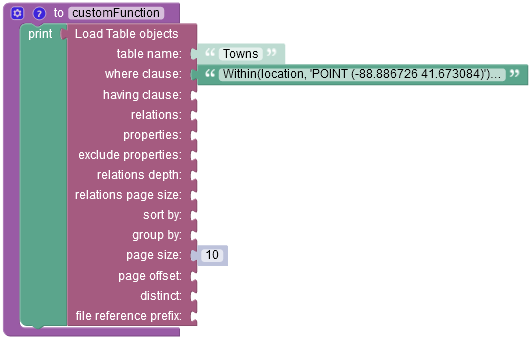The result of this operation will look as shown below after the Codeless logic runs. The object returned in the response matches the where clause condition.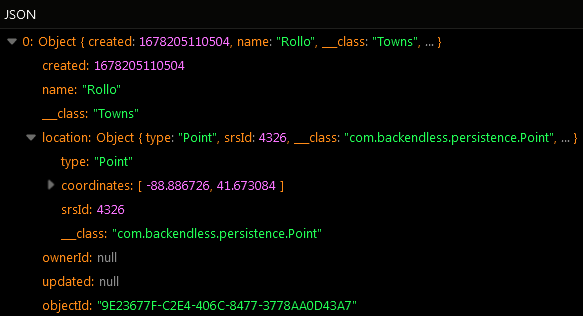### Contains Operator¶

This operator retrieves all data objects whose values for the specified spatial type column complete contain the specified geometry. This operator performs the retrieval in the opposite relationship as `Within` (see above).

Syntax:

``````Contains( spatialColumnName, 'Geometry as WKT' ) = Condition
``````

where:

Argument                Description
`spatialColumnName` Name of a spatial type column in the data table where the data objects are retrieved from; Returned objects have values in the `spatialColumnName` column which contain the geometry identified by `'Geometry as WKT'`.
`'Geometry as WKT'` A WKT string (must be enclosed into single quotes) which designates the boundary of a shape which must be contained within the values from the `spatialColumnName` column;
`Condition` `True` or `False`

Examples:

Retrieve all data objects where the geometries in the `visitedPlaces` column contain the specified point:

``````Contains( visitedPlaces, 'POINT(-116.396023 36.643763)') = True
``````

Codeless Reference

Consider the following locations(`visitedPlaces` column) in the `userGeolocation` data table: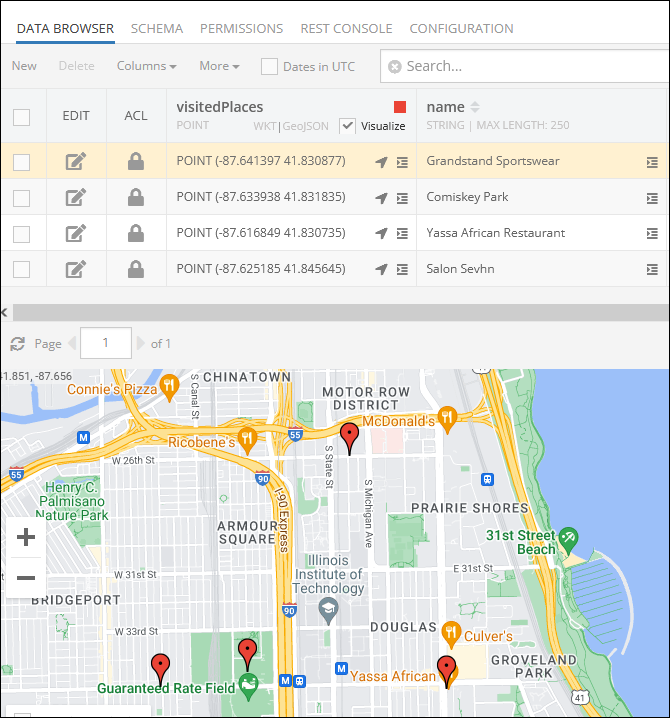The example below retrieves all data objects where the geometries in the `visitedPlaces` column contain the specified point:

``````Contains( visitedPlaces, 'POINT (-87.616849 41.830735)') = True
``````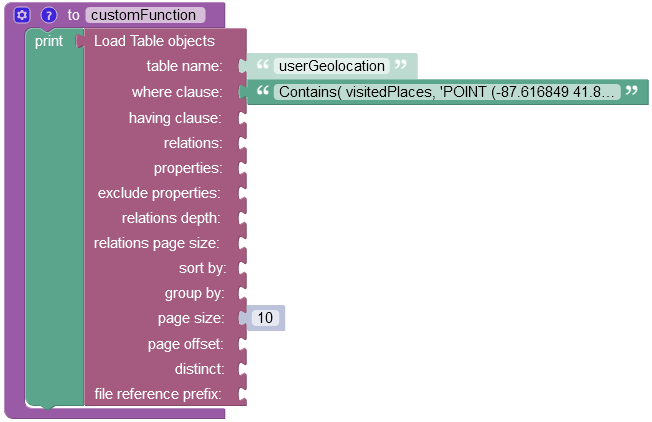The result of this operation will look as shown below after the Codeless logic runs. The object returned in the response matches the where clause condition.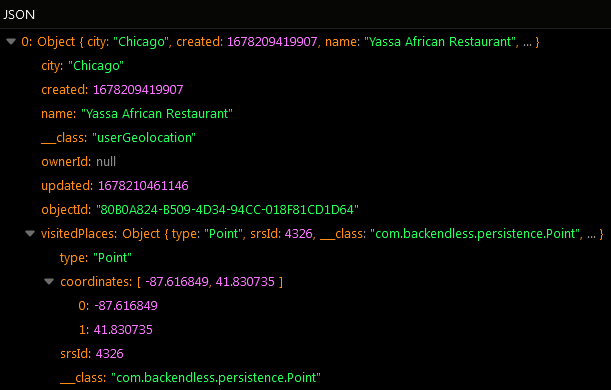### distanceOnSphere Operator¶

This operator returns the minimal spherical distance between the `POINT` values in the column (first argument) and the `POINT` value in the second argument. This operator can be used to effectively perform a search in radius. The operator returns the value in meters. Backendless identifies all objects whose values for the specified column match the condition.

Syntax:

``````// search in radius - calculates the distance between the value in the column (first argument) and the point in the second argument
distanceOnSphere( spatialColumnName, 'POINT as WKT' )

// calculate the distance between the point in spatialColumnNameFrom and the point in spatialColumnNameTo
distanceOnSphere( spatialColumnNameFrom, spatialColumnNameTo )
``````

where:

Argument                Description
`spatialColumnName` Name of a column of type `POINT`. The return value is the distance in meters between the value in the `spatialColumnName` column and the point specified in the second argument
`'POINT as WKT'` A WKT string (must be enclosed into single quotes) which designates a `POINT` for which Backendless will calculate the distance on sphere. The distance is calculated between this point and the `POINT` values stored in `spatialColumnName`.
`spatialColumnNameFrom` Name of a column of type `POINT`. The return value is the distance in meters between the value in this column and the value in the same object/record from the `spatialColumnNameTo` column.
`spatialColumnNameTo` Name of a column of type `POINT`. The return value is the distance in meters between the value in this column and the value in the same object/record from the `spatialColumnNameFrom` column.

Examples:

Retrieve all data objects whose values in the `location` column are located within 1000 meters from a point with coordinates `-96.823563 33.077570`:

``````distanceOnSphere(location, 'POINT(-96.823563 33.077570)') <= 1000
``````

Retrieve all data objects where the distance between the values in the `pickupLocation` and the `dropoffLocation` columns is less than 1000 meters:

``````distanceOnSphere(pickupLocation, dropoffLocation) <= 1000
``````

Codeless Reference

Consider the following records and locations in the `Towns` data table:The example below retrieves all data objects whose values in the `location` column are located within 9000 meters from a point with coordinates `-88.839715 41.581650`:

``````distanceOnSphere(location, 'POINT(-88.839715 41.581650)') <= 9000
``````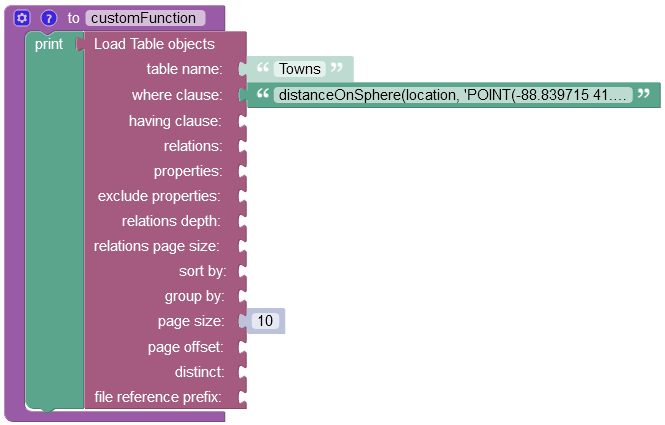The result of this operation will look as shown below after the Codeless logic runs. The operation has returned objects representing two towns located within 9000 meters from the specified coordinates point.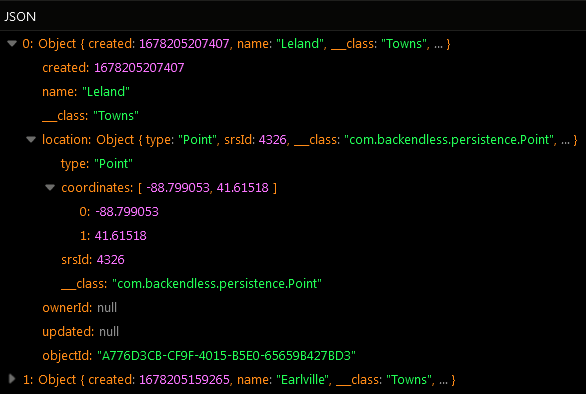### GetX¶

This operator returns the `X` coordinate (the `longitude`) for the `POINT` values stored in the specified column.

Syntax:

``````GetX( spatialColumnName ) op Value
``````

where:

Argument                Description
`spatialColumnName` Name of a column of type `POINT`. Returned objects have `X`/`longitude` values in the `spatialColumnName` column which satisfy the condition expressed via `op Value`.
`op` one of the following comparison operators:
`>` greater than
`<` less than
`>=` greater than or equals
`<=` less than or equals
`=` equals
`!=` not equals
`Value` a numeric value to compare the return value of the operator with.

Examples:

Retrieve all data objects whose longitude values in the `location` column are in the range between 41.92 and 44.66 (the continental United States):

``````GetX(location) > 41.92 && GetX(location) < 44.66
``````

Codeless Reference

Consider the following locations in the `Cities` data table: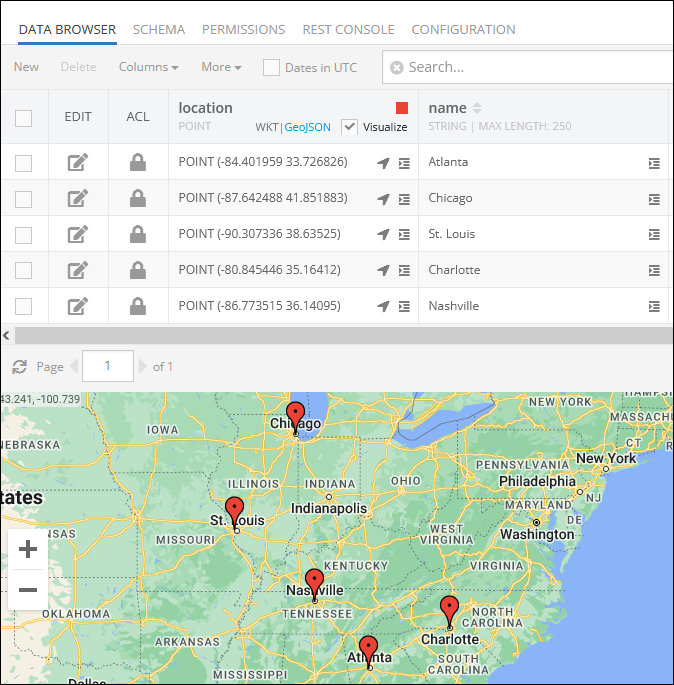The example below retrieves all data objects whose longitude values in the `location` column are in the range between `-80.27` and `-88.10` (the continental United States):

``````GetX(location) > -80.27 && GetX(location) < -88.10
``````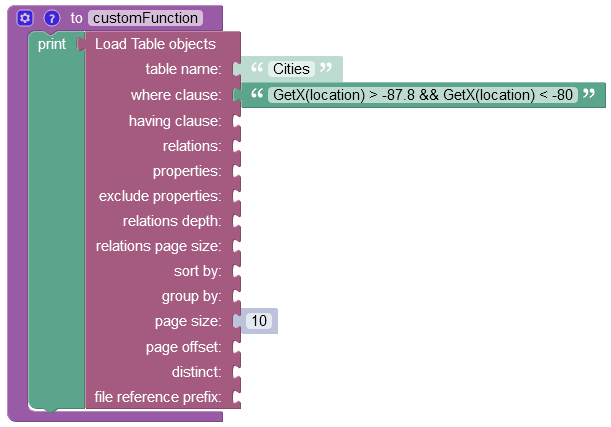After the Codeless logic runs, the operation returns four objects representing cities whose longitude match the where clause condition.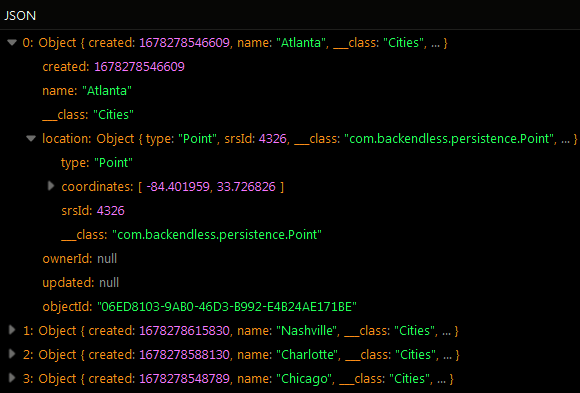### GetY¶

This operator returns the y coordinate (the `latitude`) for the `POINT` values stored in the specified column.

Syntax:

``````GetY( spatialColumnName ) op Value
``````

where:

Argument                Description
`spatialColumnName` Name of a column of type `POINT`. Returned objects have `Y`/`latitude` values in the `spatialColumnName` column which satisfy the condition expressed via `op Value`.
`op` one of the following comparison operators:
`>` greater than
`<` less than
`>=` greater than or equals
`<=` less than or equals
`=` equals
`!=` not equals
`Value` a numeric value to compare the return value of the operator with.

Examples:

Retrieve all data objects whose latitude values in the `location` column are in the range between -124.27 and -117.12 (the continental United States):

``````GetY(location) > -127.27 && GetY(location) < -117.12
``````

Codeless Reference

Consider the following locations in the `Cities` data table:The example below retrieves all data objects whose latitude values in the `location` column are in the range between `32.20` and `38.15` (the continental United States):

``````GetY(location) > 32.20 && GetY(location) < 38.15
``````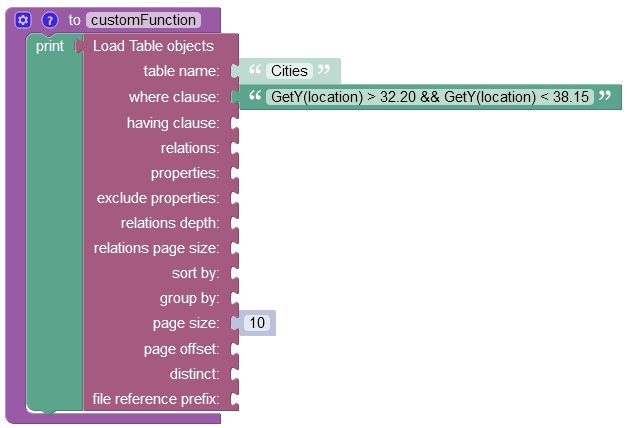After the Codeless logic runs, the operation returns three objects representing cities whose latitude match the where clause condition.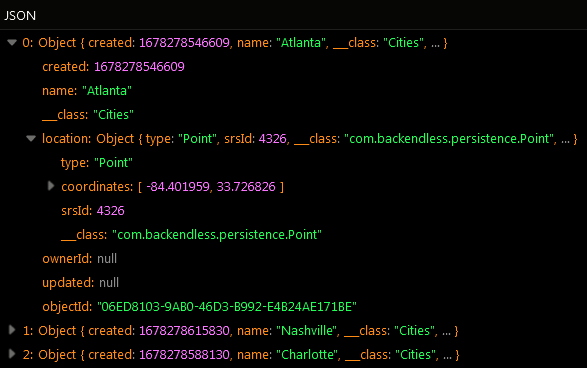### AsWKT¶

This operator returns the WKT representation for the values stored in the specified column.

Syntax:

``````AsWKT(spatialColumnName) op Value
``````

where:

Argument                Description
`spatialColumnName` Name of a column values of which will be used to obtain the WKT representation from.
`op` one of the following comparison operators:
`=` equals
`!=` not equals
`Value` a string value to compare the return value of the operator with.

Examples:

Retrieve all data objects which match the specified WKT representation

``````AsWKT(location) = 'POINT(-124.27 41.92)'
``````

Codeless Reference

Consider the following locations in the `Cities` data table:The example below retrieves the data object whose longitude and latitude values in the `location` column are `-84.401959 33.726826` (the continental United States):

``````AsWKT(location) = 'POINT(-84.401959 33.726826)'
``````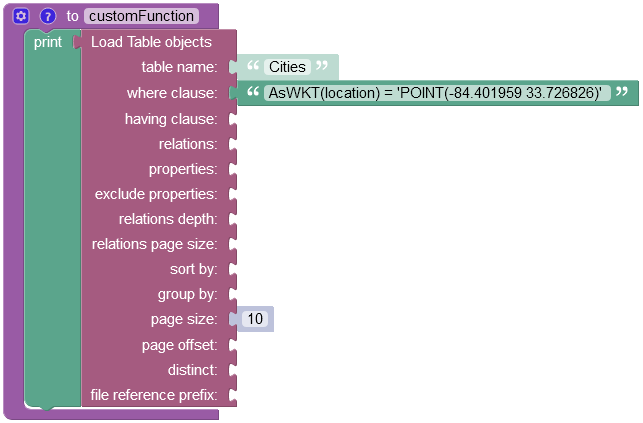After the Codeless logic runs, the operation returns one object whose longitude and latitude match the where clause condition.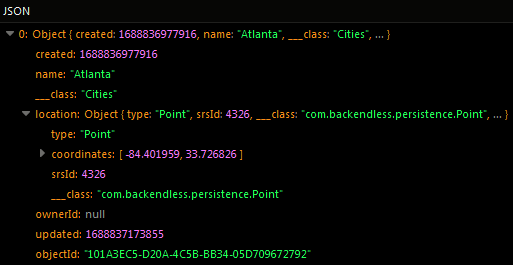### AsGeoJSON¶

This operator returns the GeoJSON representation for the values stored in the specified column.

Syntax:

``````AsGeoJSON(spatialColumnName) op Value
``````

where:

Argument                Description
`spatialColumnName` Name of a column values of which will be used to obtain the GeoJSON representation from.
`op` one of the following comparison operators:
`=` equals
`!=` not equals
`Value` a string value to compare the return value of the operator with.

Examples:

Retrieve all data objects which match the specified GeoJSON representation

``````AsGeoJSON(location) = 'TBD'
``````